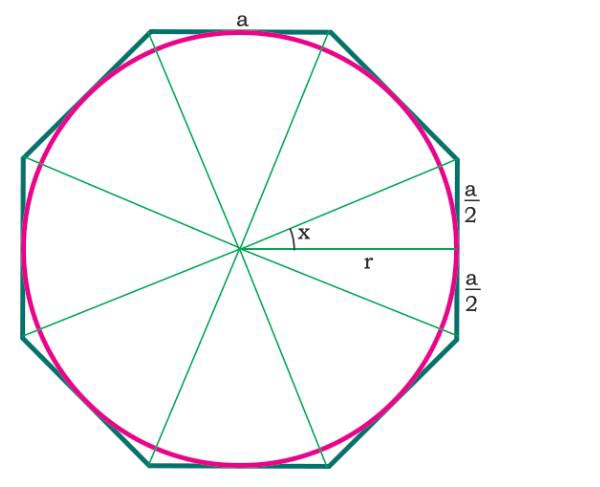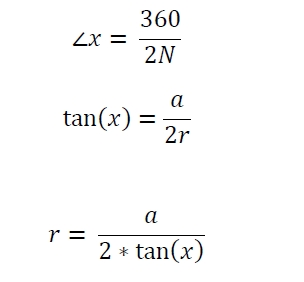# Area of largest Circle inscribe in N-sided Regular polygon in C Program?

Here we will see how to get the area of the circle which is inscribed in N-sided regular polygon. The N (number of sides) are given, and each side of the polygon is ‘a’The approach is simple. One N sided polygon can be divided into N equal triangles, the whole angle for each triangle in center is 360/N, so −## Example

#include <iostream>
#include <cmath>
using namespace std;
float area(float n, float a) {
if (n < 0 || a < 0 ) //if the valuse are negative it is invalid
return -1;
float r = a/(2.0*tan((180/n) * 3.14159/180));
float area = 3.14159 * r*r;
return area;
}
int main() {
float n = 8, a = 4;
cout << "Area : " << area(n, a);
}

## Output

Area : 73.2422